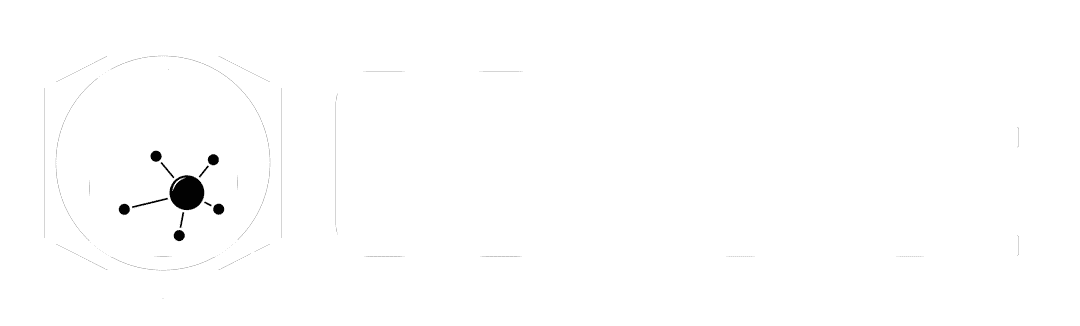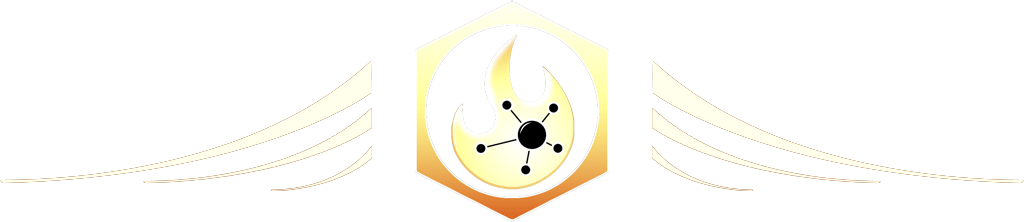# Scatter

The Ultimate Houdini node reference

# Visit the Node Bible

### Scatter

#### The Scatter SOP will generate points based on the incoming geometry.

Drag to resize

Summary:

The Scatter SOP gets used all the time when sourcing information into DOPS, creating points along a surface which are used for instancing, and much more.  Most of the time, the default settings will allow you to generate points for whatever situation you need.  However, it is good to know about relax iterations, different ways of generating the scatter, and integrating the density attribute for better control of where points are spawned.  Keep in mind that if you source in a fog volume, this node will spawn points within the fog volume.

Drag to resize

Main Parameters:

Generate:

--  By Density:

*  "Points will be distributed independently based on having a density over each primitive. Density is the expected number of points per length unit for curves, per area unit for surfaces, and per volume unit for volumes and tetrahedra."  In other words, it looks at the area, length, or volume value for determining where points are scattered.  The "density" variable is then calculated based on how many points you want with the Force Total Count and the area, length, or volume value of the associated primitive.  Keep in mind that if you're using a volume, increasing the density of the volume will not increase the number of points which are scattered there.  This only determines where points are allowed to scatter - which is wherever the values are positive.

--  Count Per Primitive:

*  This setting relies on an integer primitive attribute called "count" by default.  This attribute will control how many points are scattered along each primitive based on that attribute value.

--  In Texture Space:

*  This allows you to control how many points are scattered based on the scale of uv islands.  What this means is that if you scale up a UV island, then more points will be scattered there.  If you scale down a uv island, then less points will be scattered there.  This is super-useful when, as an example, you're trying to scatter points along terrain and you want certain areas to receive more or less points.

Density Scale:

--  If you check on "Scatter on Voxels Independently" then you can manually adjust the amount of points with this setting.  The "Scatter on Voxels Independently" basically allows you to add more points based on the @density attribute value.  In other words, without this checked on, the "Force total count" will determine how many points are scattered in a volume.  With "Scatter on Voxels Independently" the voxel values will now be able to scatter more points based on the voxel values.

Density Attribute:

--  This allows you to specify an attribute which will control how many points are scattered along the surface.  This attribute can be a point, vertex, primitive, or detail attribute.  If "Force Total Count" is on, then this determines how likely a point is to spawn there - not how many points are generated.  If "Scatter on Voxels Independently" is turned on, then the number of generated points will scale up with the attribute value.

Override Prim Area:

-- This setting relies on using the @area attribute, and acts pretty much just like the Density Attribute described above.  The Area attribute gets used along with the force total count to determine how many points are scattered somewhere.  If you want to scale up the @area attribute, then that will act like a multiplier to the Force Total Count.  In practice, this lets you adjust @area for determining how many points are generated, and you don't need to use "Scatter on Voxels Independently" to scale things up while using an attribute.

Use Area for 2D Volumes:

-- This setting is only available when using "Scatter on Voxels Independently."  If you happen to be using any 2D Volumes, then the scatter node will use the @area attribute as a multiplier against @density to determine how many points are scattered.  A 2D volume is a volume which does not contain x,y,z coordinates.  It only contains two of the three dimensions.  In other words, it's like an infinitely flat plane of voxels.  The @area attribute can be created as a primitive attribute, and in practice, this offers you multiplier to the number of scattered points.

Force Total Count:

--  Determines exactly how many points are to be scattered.

Density Texture:

--  This parameter allows you to use a texture map when determining how many points you'd like to scatter.  This is only available when setting the "Generate" parameter to "In Texture Space."

Count per Prim Attribute:

--  See above in the "Generate" section under "Count Per Primitive"

Max Points:

--  Sets a maximum number of scattered points, (which is useful for times when you don't want to crash Houdini by accident)

Primitive Seed Attribute:

--  When the "Generate" is set to "By Density," it will look at each primitive and determine how things scatter based on the primitive id.  Enabling the "Primitive Seed Attribute" will force the scatter to look at an attribute value for the seed instead.

In practice, here's where this is useful.  As an example, lets say that you want to scatter points along debris and you want the scattering pattern to stay the same for each debris piece.  By default, the scatter looks at the prim id as a seed for how it should scatter.  That's a problem if you ever want to spawn additional debris because that would then change the prim ids.  Remember, prim id = seed by default.  So, instead, you check on the "Primitive Seed Attribute" to determine the seed using an attribute.  In this situation you could then use the connectivity node to create a "class" attribute for each debris piece, and set that class to the prim seed attribute so that the scattering stays the same on each piece.  That's a situation where you'll really love this checkbox.

Randomize Point Order

--  If checked off, then the point ids are assigned based on their position.  If turned on, then the point ids are randomized.  According to the docs, "When enabled, the points will be in random order in the output detail. The disadvantage is that if more points are generated in one area, it will change the order of points in other areas, even though the positions and attributes may stay consistent."  In practice, if you want to keep the point ids based on position, then check this off.

Relax Iterations

--  Relax iterations will make the spacing of the scattered points more evenly distant from each other. More iterations = more accurate distance away from each point but also slows things down.

-- When the points are spread out from each other, they take an imaginary sphere ("Radii") And if another point falls inside the radius, then it pushes away from that particle. You can specify this radii size here. It's also good to note that going negative will clump the particles together.

-- This gets used for technical reasons - basically to prevent a point from searching out into infinity for points to relax with. If you turn this off, the maximum distance a point can look is half of the diagonal of the bounding box of the object. So, a value of 0 ought to suffice for nearly all situations. If you're interested in the technical reasons why this is the case, then check out the user doc text:
"This must be set appropriately to prevent outlier points in low-density areas causing problems when relaxing points.
In order to approximately maintain variations in density, points are assigned radii inversely proportional to density (for curves), the square root of density (for surfaces), or the cube root of density (for volumes). This may cause problems for areas whose densities are near zero, especially in volumes, when painting density on a surface, or when using a density texture with a black background, since the radius approaches infinity. This parameter specifies the maximum radius within which points will influence other points.
When this is disabled, there will still be a maximum radius, which is currently chosen as half the diagonal length of the bounding box of the input geometry."

Drag to resize

Output Attributes:

-----------------

In this section, you can tell the scatter sop which attributes you want to have on your points.  Attributes are picked up from the geometry that you scattered the points on.  If you decide to toggle on "Output radius and density in texture space," then it will use the distances based on uv space rather than world space.  You'll also need to have the "Generate" set to "In Texture Space" for this checkbox to become available.
-----------------